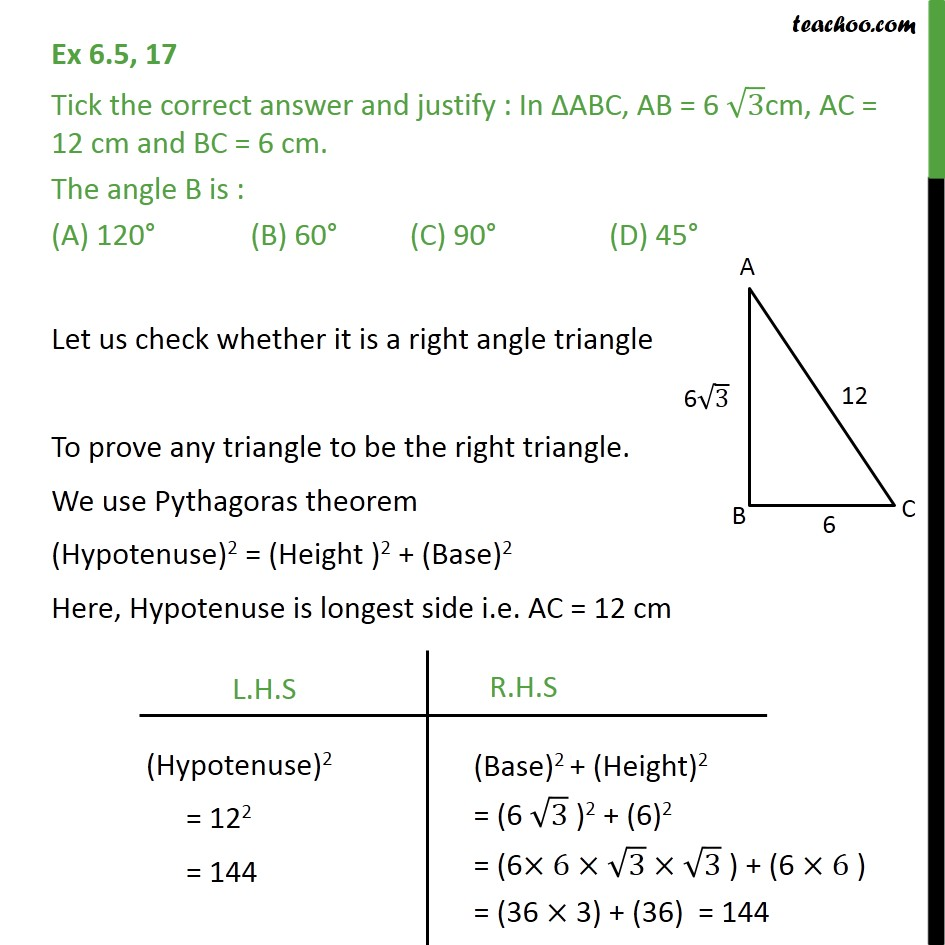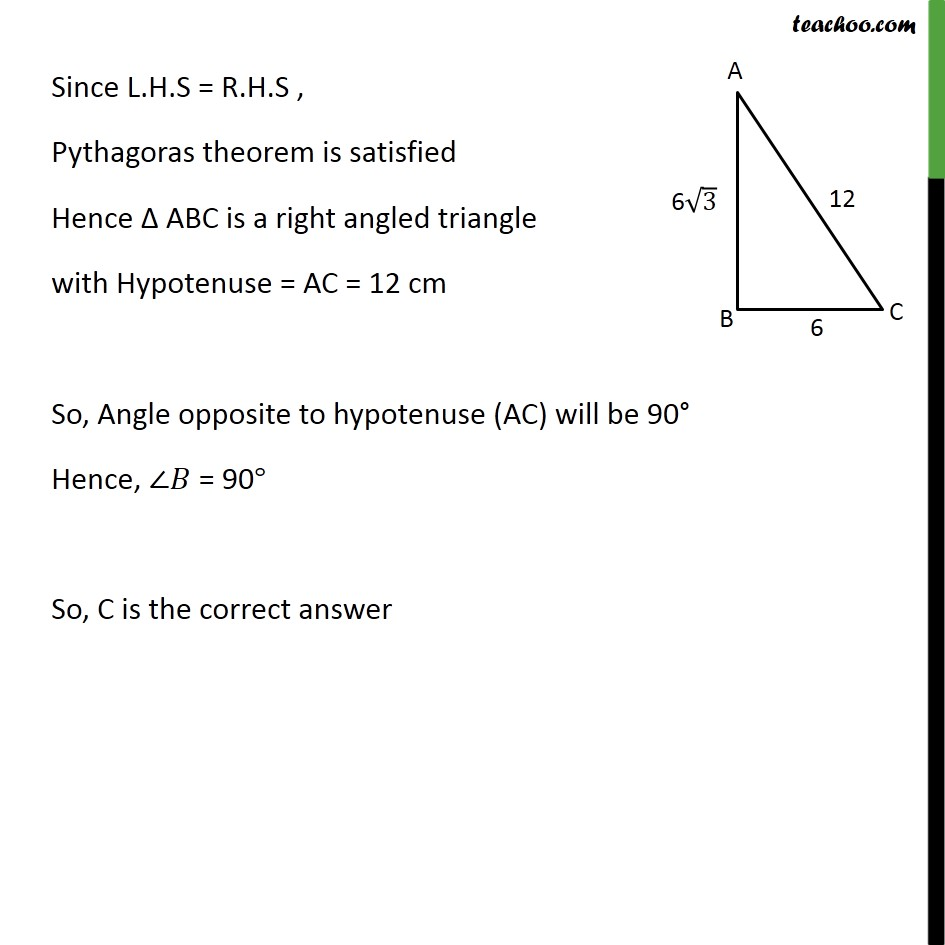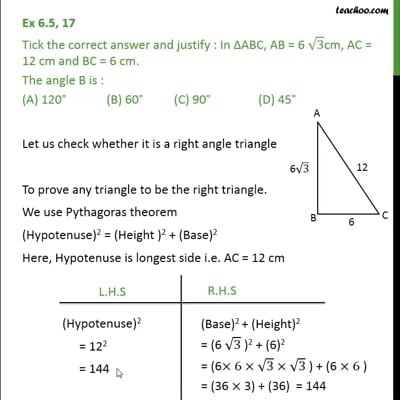Ex 6.5

Chapter 6 Class 10 Triangles
Serial order wiseThis video is only available for Teachoo black users

Maths Crash Course - Live lectures + all videos + Real time Doubt solving!

### Transcript

Ex 6.5, 17 Tick the correct answer and justify : In ABC, AB = 6 3cm, AC = 12 cm and BC = 6 cm. The angle B is : (A) 120 (B) 60 (C) 90 (D) 45 Let us check whether it is a right angle triangle To prove any triangle to be the right triangle. We use Pythagoras theorem (Hypotenuse)2 = (Height )2 + (Base)2 Here, Hypotenuse is longest side i.e. AC = 12 cm Since L.H.S = R.H.S , Pythagoras theorem is satisfied Hence ABC is a right angled triangle with Hypotenuse = AC = 12 cm So, Angle opposite to hypotenuse (AC) will be 90 Hence, = 90 So, C is the correct answer